## 1. Setting

Reddit.com, as with any other online community, has its own “personality” resulting from the aggregated interactions of all the users that participate in the community. Anyone may post a link, but everyone else in the community can “upvote” or “downvote” the link. The best-rated links make it to the front page for all to see. Some posts are universally liked, while others are more contentious. The objective of this study is to look at successful Reddit submissions and the percent of total votes that were upvotes, indicating whether or not the community’s response to the submission was polarized.

### System under test

Github user umbrae took the top 2.5 million Reddit posts and placed them on Github as .csv files organized by subreddit (for those less familiar with Reddit, the community is subdivided into smaller topic-based communities called “subreddits”).
The chosen subreddits were r/science, r/politics, and r/news. The research hypothesis was that the percent of upvotes of the top-rated posts varies among each of these sub-communities.

### Factors and levels

The two 3-level independent variables (IVs) selected were:

• subreddit: r/science, r/politics, and r/news
• link domain: .org, .com, or other (such as .gov or .co.uk)

The two 2-level IVS selected were:

• relative number of comments: few (bottom 50%), or many (top 50%)
• time of post: earlier (before the cutoff date), or later (after the cutoff date),

### Continuous variables

The only desired continuous variable is the response variable, which is explained in the next section. However, two continuous IVs were cast as 2-level categorical variables to meet the requirements of the experimental design. Categorizing these variables into two levels was performed by sorting based on an arbitrary cutoff value. To maximize the number of available combinations of each type, the median was chosen as the cutoff value. The cutoff value between many/few comments was the median relative percent of comments (number of comments divided by total votes). The cutoff value for time of post was the median UTC code of the post time.

### Response variable

The response variable, % upvotes, was calculated for each post simply by taking the number of upvotes for a given post and dividing by the total number of votes for that post. A universally-liked post would approach 100% upvotes, while a more polarizing post would be closer to 50%.

### The Data

The raw data for each of the three subreddits of interest was imported directly from the Github repository using the RCurl library. Columns not of interest to the analysis were removed using a user-defined function called DropColumns. The three dataframes were stitched together using the rbind function. See the Appendix for full code.

Additionally, columns were added to normalize the comments (as a percentage of total votes), as well as to convert the domain type to a 3-level factor (.com, .org, and other). Finally, our response variable percent_upvotes was defined (upvotes as a percentage of total votes). The structure, head, and tail of the raw data frame (after removing and adding columns) is given below.

## 'data.frame':    2998 obs. of  10 variables:
##  $created_utc : num 1.34e+09 1.34e+09 1.35e+09 1.34e+09 1.36e+09 ... ##$ score           : int  5785 5593 4626 4486 4465 4438 4305 4066 4047 4025 ...
##  $domain : chr "twitter.com" "webcast.web.cern.ch" "nasa.gov" "guardian.co.uk" ... ##$ ups             : int  53727 33456 42063 18267 33283 9418 16929 16996 19220 12772 ...
##  $downs : int 47942 27863 37437 13781 28818 4980 12624 12930 15173 8747 ... ##$ num_comments    : int  4567 2919 1515 739 1135 1141 1165 1008 717 1069 ...
##  $subreddit_name : chr "science" "science" "science" "science" ... ##$ percent_comments: num  4.49 4.76 1.91 2.31 1.83 ...
##  $domain_type : chr ".com" "other" "other" "other" ... ##$ percent_upvotes : num  52.8 54.6 52.9 57 53.6 ...
##   created_utc score              domain   ups downs num_comments
## 1  1344231209  5785         twitter.com 53727 47942         4567
## 2  1341387530  5593 webcast.web.cern.ch 33456 27863         2919
## 3  1354217414  4626            nasa.gov 42063 37437         1515
## 4  1342384318  4486      guardian.co.uk 18267 13781          739
## 5  1361725918  4465         reuters.com 33283 28818         1135
## 6  1363051689  4438          nature.com  9418  4980         1141
## 1        science         4.492028        .com        52.84502
## 2        science         4.760352       other        54.56058
## 3        science         1.905660       other        52.90943
## 4        science         2.305916       other        56.99888
## 5        science         1.827668        .com        53.59495
## 6        science         7.924712        .com        65.41186
##      created_utc score                domain  ups downs num_comments
## 2993  1366385781  1031           reuters.com 1439   408          157
## 2994  1364274310  1033 earthquake-report.com 1390   357          262
## 2995  1292330835  1024     newsfeed.time.com 2282  1258          206
## 2996  1374840797  1027        guardian.co.uk 1390   363           35
## 2997  1342784992  1023             self.news 1862   839          442
## 2998  1376504442   809            nbcdfw.com 1052   243          176
## 2993           news         8.500271        .com        77.91012
## 2994           news        14.997138        .com        79.56497
## 2995           news         5.819209        .com        64.46328
## 2996           news         1.996577       other        79.29264
## 2997           news        16.364310       other        68.93743
## 2998           news        13.590734        .com        81.23552

## 2. (Experimental) Design

The objective of this report is to perform a Fractional Factorial Design. First, a full factorial design was created of the form $$2^{2}*3^{2}$$, and then 3-level factors were broken down each into 2 2-level factors to create a $$2^{6}$$ full factorial design. From this last design, a $$2^{m-3}$$ design could be generated.

### Organization of experiment to test the hypothesis

The experiment is organized such that we are performing ANOVA on a linear model developed from a fractional factorial design. Since the design and analysis have occured after the data was collected, the sample was selected based on groups in order to provide a balanced design.

#### Full factorial design framework

The $$3^{2}*2^{2}$$ full factorial design requires two 3-level categorical IVs and two 2-level categorical IVs. The 3-level IVs (subreddit, domain type) are inherently categorical. The 2-level IVs (relative number of comments, time posted) are continuous but were allocated into categories based on cutoff values defined by the median of the respective columns. The median was selected to provide the most even portioning of the dataset in order to maximize the number of potential replicates.

A $$3^{2}*2^{2}$$ full factorial design has 36 possible combinations. Each of these possible combinations can be assigned an experiment code (0 through 35) based on the following tree-like sorting structure (Fig. 1):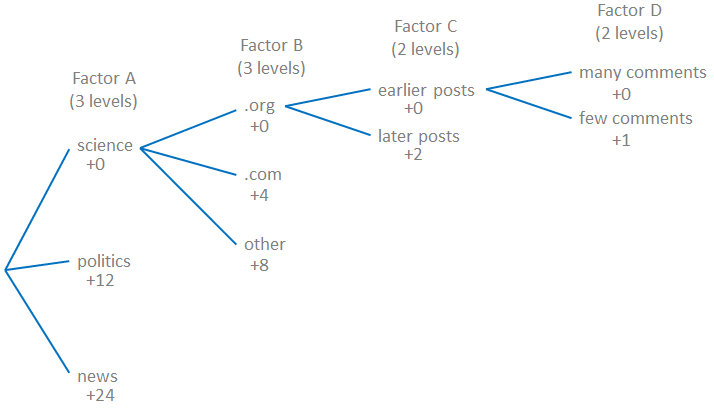Fig. 1 Sorting structure to determine experiment codes for each combination in the $$3^{2}*2^{2}$$ full factorial design.

By applying the experiment code assignment to each row in the data frame, a balanced sample selection becomes simpler because one only needs to make sure that an equal number of each experiment code is represented in the sample. The maximum number of replicates is therefore determined by the least-occurring experiment code.

A histogram of the experiment codes shows that all possible factor-level combinations exist within the data frame (Fig. 2). The least-occurring experiment code has 8 occurrences, meaning that we could perform up to 8 replicates of the full factorial experiment.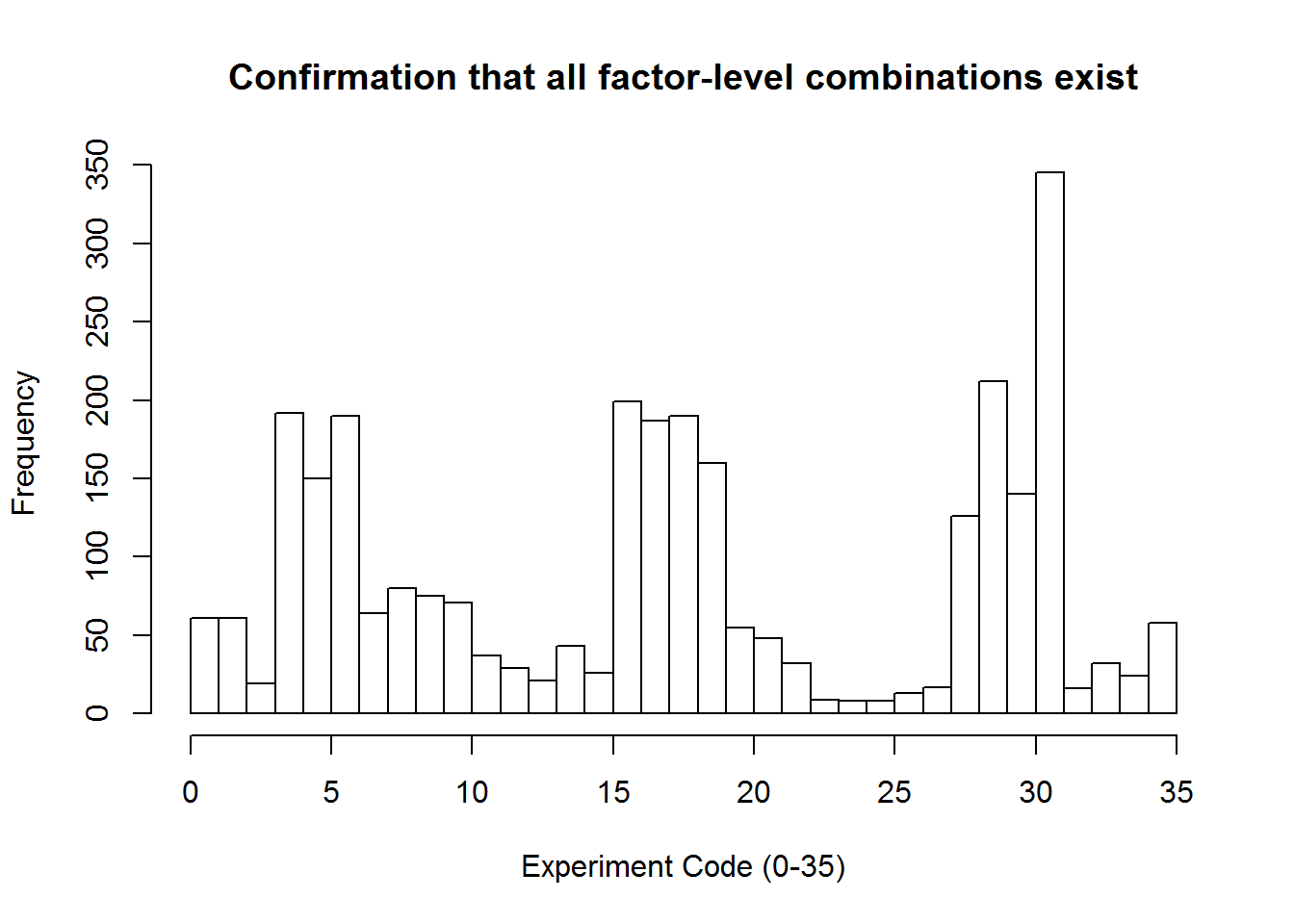Fig. 2 Distribution of experiment codes for the $$3^{2}*2^{2}$$ design indicates that we have at least one of each possible factor-level combination to run an experiment.

#### Representing 3-level factors as 2-level factors

Montgomery Table 9.9 provides a breakdown for using two 2-level factors to form a 3-level factor.1 In this report, we are taking the opposite approach and creating two 2-level factors from a 3-level factor. While the highest and lowest levels are explicitly defined in this structure, the middle-level of a 3-level is not uniquely defined and can take either the (1,-1) or (-1,1) arrangement.

Neither of the 3-level factors is ordinal, therefore dividing these factors into two 2-level factors has no physical meaning.

Subreddit (3-level) was broken down into SR1 and SR2, using the following criteria:

• r/science: (SR1, SR2) = (-1, -1)
• r/politics: (SR1, SR2) = (-1, 1) or (1, -1) [alternated assignment]
• r/news: (SR1, SR2) = (1, 1)

Domain type (3-level) was broken down into DT1 and DT2, using the following criteria:

• .org: (DT1, DT2) = (-1, -1)
• .com: (DT1, DT2) = (-1, 1) or (1, -1) [alternated assignment]
• other: (DT1, DT2) = (1, 1)

Two boolean variables were created, SR_toggle and DT_toggle, to toggle between the (1,-1) and (1,-1) combinations for the respective middle-levels.

A similar coding scheme was applied to generate experiment codes for the $$2^{6}$$ design, this time with 64 possible combinations (0-63). After sorting, a histogram was inspected to confirm that all possible factor-level combinations were represented in the design (Fig. 3). In this case, the least-occurring combination has a frequency of 4, indicating that we can include up to 4 replicates in our sample.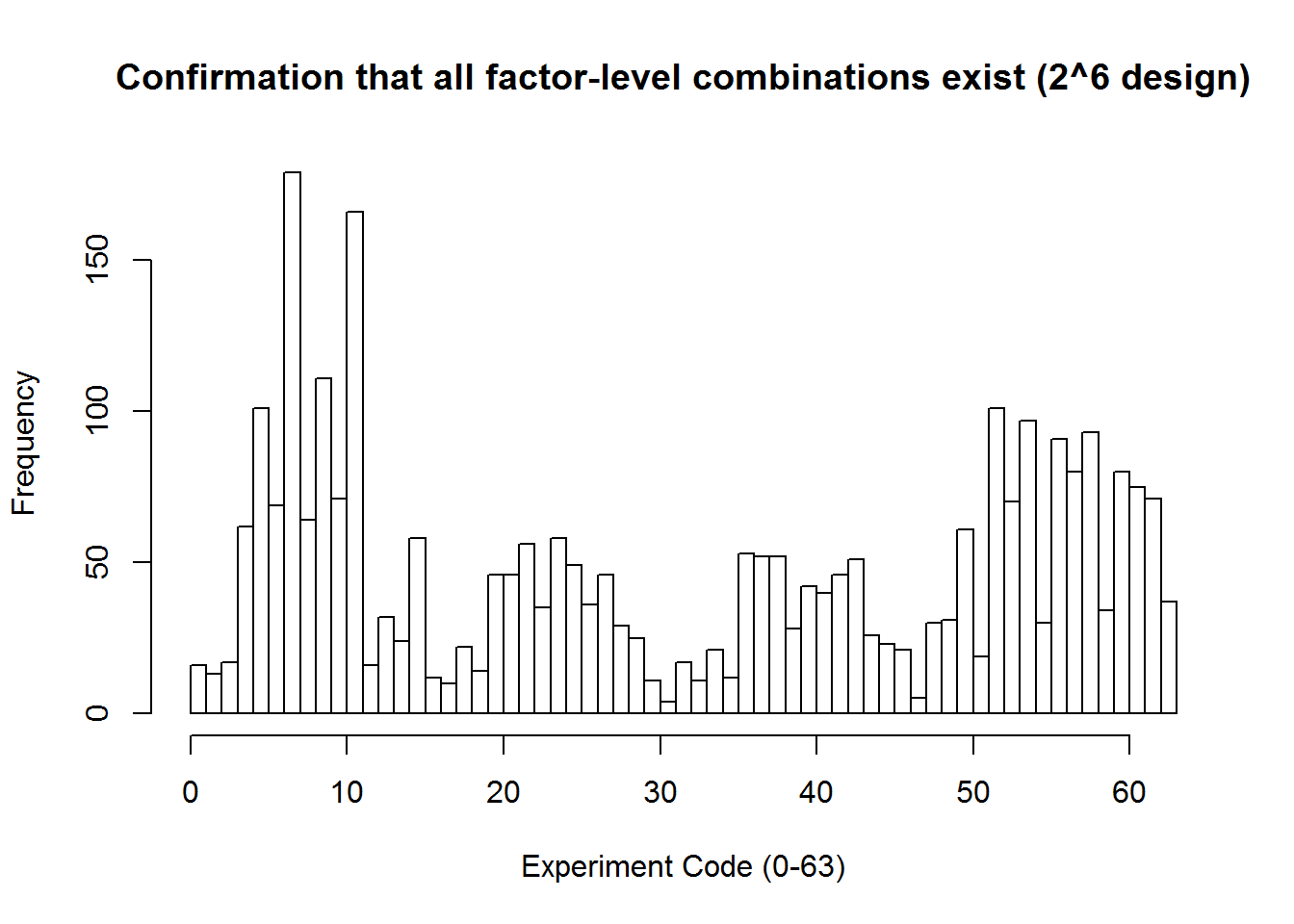Fig. 3 Distribution of experiment codes for $$2^{6}$$ design indicates that we have at least one of each possible factor-level combination.

Finally, we need to generate a sample of the data frame that “collects” an equal number of each possible combination. Functions provided within the dplyr package enable us to achieve such sampling by group. Here, the group_by() function was applied to the experiment code column. The size of sample_n() was determined based on the frequency of the least-occurring experiment code. Therefore, this approach maximizes the number of replicates for a given dataset, assuming that there is at least 1 of each combination.

# set RNG seed (for reproducible results)
set.seed(1)

# create the sample
my_sample2 <- all_data2 %>% group_by(exp_code) %>% sample_n(size = min(exp_table2))

# set appropriate columns as factors, and return subreddit_name and domain_type to characters
all_data2$SR1 <- as.factor(all_data2$SR1)
all_data2$SR2 <- as.factor(all_data2$SR2)
all_data2$DT1 <- as.factor(all_data2$DT1)
all_data2$DT2 <- as.factor(all_data2$DT2)
all_data2$time_code <- as.factor(all_data2$time_code)
all_data2$comments_code <- as.factor(all_data2$comments_code)
all_data2$subreddit_name <- as.character(all_data2$subreddit_name)
all_data2$domain_type <- as.character(all_data2$domain_type)

head(my_sample2)
## Source: local data frame [6 x 17]
## Groups: exp_code 
##
##   created_utc score            domain   ups downs num_comments
##         <dbl> <int>             <chr> <int> <int>        <int>
## 1  1348344228  2034     hosted.ap.org  3770  1736          230
## 2  1330708319  1156 addictinginfo.org  1885   729          123
## 3  1352389144  1734           npr.org  2521   787          180
## 4  1326295588  1694    baycitizen.org  3117  1423          127
## 5  1330302687  1452     wikileaks.org  2328   876          236
## 6  1340833971  1125           npr.org  1791   666          383
## # ... with 11 more variables: subreddit_name <chr>,
## #   time_code <dbl>, comments_code <dbl>, DT1 <dbl>, DT2 <dbl>, SR1 <dbl>,
## #   SR2 <dbl>, exp_code <dbl>
tail(my_sample2)
## Source: local data frame [6 x 17]
## Groups: exp_code 
##
##   created_utc score            domain   ups downs num_comments
##         <dbl> <int>             <chr> <int> <int>        <int>
## 1  1366163844  2038 news.stanford.edu  4031  1993          340
## 2  1359746821  1868      concordia.ca  4123  2255          276
## 3  1367435317  2317    guardian.co.uk  5618  3301          855
## 4  1358872643  2297   money.aol.co.uk 11879  9582         1954
## 5  1372681963  2430    imperial.ac.uk 10226  7796         2207
## 6  1364178841  2874     thejournal.ie 15975 13101         2165
## # ... with 11 more variables: subreddit_name <chr>,
## #   time_code <dbl>, comments_code <dbl>, DT1 <dbl>, DT2 <dbl>, SR1 <dbl>,
## #   SR2 <dbl>, exp_code <dbl>

#### Fractional factorial design to test

A $$2^{6-3}$$ fractional factorial design requires only 8 runs (times the number of replicates) instead of the 64 required for a full factorial, leading to a 1/8 fractional design. According to Montgomery Table 8.14, the highest possible resolution for a $$2^{6-3}$$ design is Resolution III.1 The corresponding design generators for this $$2_{III}^{6-3}$$ design are $$D=AB$$, $$E=AC$$, and $$F=BC$$. In order to achieve a higher resolution, we would need 16 runs for a $$2_{IV}^{6-2}$$ design.

### Rationale for design

The rationale for a fractional factorial design is to minimize the time and cost associated with running experiments, thus maximizing efficiency. The trade-off is that, because we are only running a fraction of all possible combinations, some information is lost in the process. However, by appropriately selecting orthogonal trials, we can isolate the lost information to higher-order interactions, which we assume are negligible anyway. More information on the aliasing structure can be found in the Summary of Relevant Theory section below.

### Randomization

Randomization is always important in experimental design, and perhaps moreso in fractional factorial designs where the extracted information is highly leveraged. Here, the dataset was organized by assigning experiment codes to each factor-level combination, and then a random sample was taken. Because the sample is ordered, it is necessary to run the experimental runs in a random order.

### Replication

Replication is not a necessity for this report, but rather a luxury if the dataset is large enough to provide multiple instances of each factor-level combination. Here, we are able to use up to 4 replicates. However, the benefit of using a fractional factorial design is somewhat lost if you run too many replicates; it might be more advantageous to run fewer replicates but more trial runs, thus increasing the resolution of the design.

### Blocking

Blocking against nuisance factors was not considered within the scope of this analysis.

## 3. (Statistical) Analysis

The R package FrF2 was used to plan out the $$2_{III}^{6-3}$$ fractional factorial design.

# create fractional factorial design using FrF2 package
plan <- FrF2(8, 6, factor.names = c("SR1", "SR2", "DT1", "DT2", "time_code", "comments_code"))
plan
##   SR1 SR2 DT1 DT2 time_code comments_code
## 1   1  -1  -1  -1        -1             1
## 2   1   1  -1   1        -1            -1
## 3  -1   1  -1  -1         1            -1
## 4  -1  -1   1   1        -1            -1
## 5   1  -1   1  -1         1            -1
## 6  -1  -1  -1   1         1             1
## 7  -1   1   1  -1        -1             1
## 8   1   1   1   1         1             1
## class=design, type= FrF2

A data frame mydesign was created from the plan. The proper experiment code (exp_code) was assigned to each combination using the same tree-like sorting algorithm used earlier for the full factorial designs.

##   SR1 SR2 DT1 DT2 time_code comments_code exp_code
## 1   1  -1  -1  -1        -1             1       33
## 2   1   1  -1   1        -1            -1       52
## 3  -1   1  -1  -1         1            -1       18
## 4  -1  -1   1   1        -1            -1       12
## 5   1  -1   1  -1         1            -1       42
## 6  -1  -1  -1   1         1             1        7
## 7  -1   1   1  -1        -1             1       25
## 8   1   1   1   1         1             1       63

A subset of the data called eligibledata was then taken, where only data matching one of the combinations in the plan were chosen. The selection was made based on the exp_code column and is.element() function. A histogram shows the eligible data from which a sample could be pulled (Fig. 4).

# create subset of eligible data that meets the criteria of the fractional factorial design (using exp_code as the filter)
eligibledata <- all_data2[is.element(all_data2$exp_code, mydesign$exp_code),]

# check spread of exp codes for all_data2
hist(eligibledata$exp_code, breaks = 64, xlab="Experiment Code (0-63)", main = "Eligible data for sampling")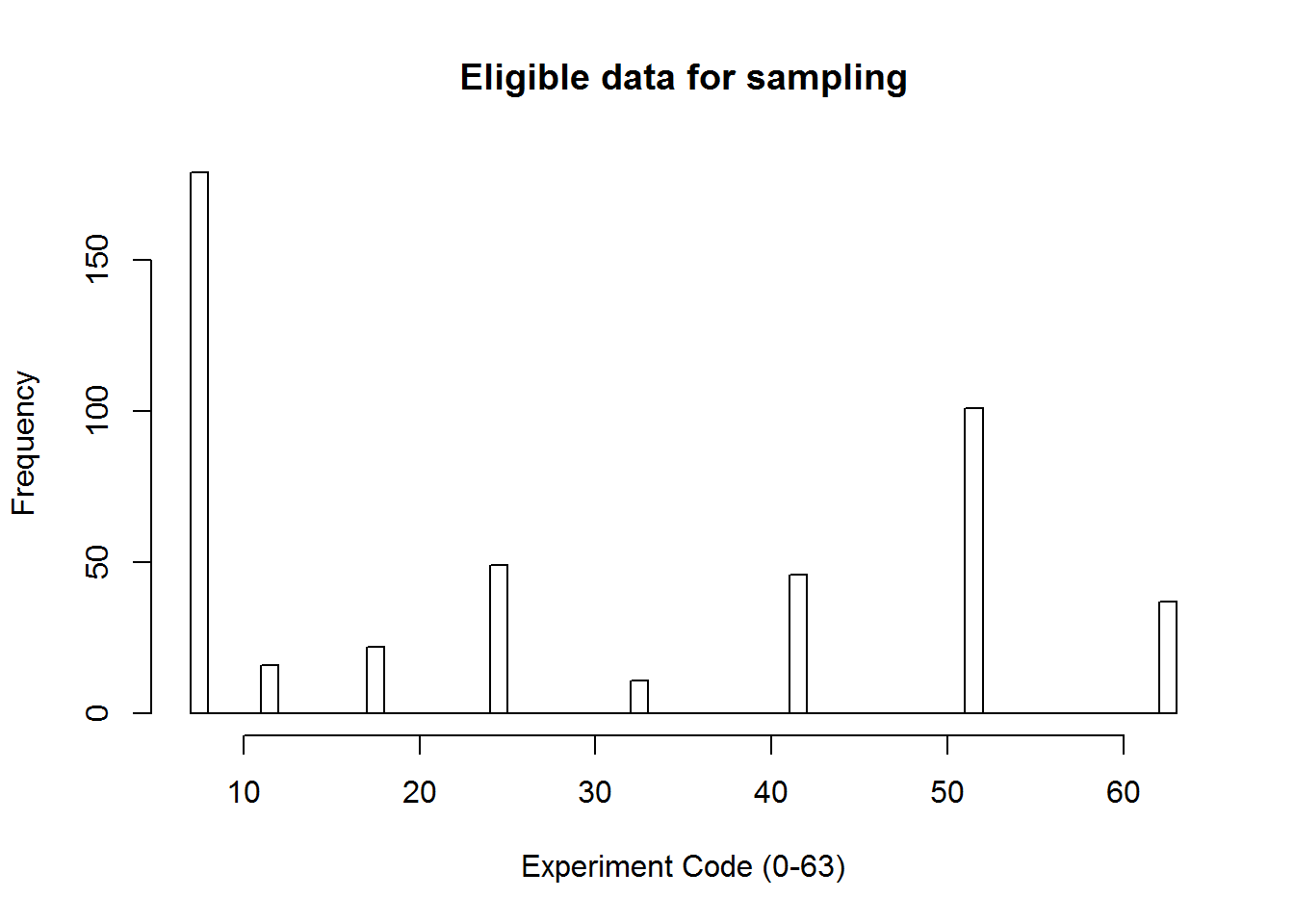Fig. 4 Distribution of eligible experiment codes for the $$2_{III}^{6-3}$$ design. A sample of this data was taken with only 1 replicate of each exp_code, giving a total of 8 runs for the $$2_{III}^{6-3}$$ fractional factorial design. The rows of the sample needed to be sorted to match the order given in the FrF2 design. A column with the response variables was then added to the FrF2 design using the add.response() function. # create a sample of the eligible data fractionaldata <- eligibledata %>% group_by(exp_code) %>% sample_n(size = 1) # show the values of the response variable (percent_upvotes) for each corresponding exp_code response <- fractionaldata[,c("exp_code", "percent_upvotes")] # re-arrange rows of the response variables so that the exp_code matches up with the mydesign dataframe response <- response[match(as.vector(mydesign$exp_code), response$exp_code),] # add column with the response variable to the FrF2 design results <- add.response(plan, as.vector(response$percent_upvotes))
summary(results)
## Call:
## FrF2(8, 6, factor.names = c("SR1", "SR2", "DT1", "DT2", "time_code",
##
## Experimental design of type  FrF2
## 8  runs
##
## Factor settings (scale ends):
##   SR1 SR2 DT1 DT2 time_code comments_code
## 1  -1  -1  -1  -1        -1            -1
## 2   1   1   1   1         1             1
##
## Responses:
##
## Design generating information:
## $legend ##  A=SR1 B=SR2 C=DT1 D=DT2 ##  E=time_code F=comments_code ## ##$generators
##  D=AB E=AC F=BC
##
##
## Alias structure:
## $main ##  A=BD=CE B=AD=CF C=AE=BF D=AB=EF E=AC=DF F=BC=DE ## ##$fi2
##  AF=BE=CD
##
##
## The design itself:
##   SR1 SR2 DT1 DT2 time_code comments_code
## 1   1  -1  -1  -1        -1             1
## 2   1   1  -1   1        -1            -1
## 3  -1   1  -1  -1         1            -1
## 4  -1  -1   1   1        -1            -1
## 5   1  -1   1  -1         1            -1
## 6  -1  -1  -1   1         1             1
## 7  -1   1   1  -1        -1             1
## 8   1   1   1   1         1             1
## 1                            64.33472
## 2                            66.83466
## 3                            54.87886
## 4                            64.37679
## 5                            56.45731
## 6                            76.59183
## 7                            57.97362
## 8                            59.15430
## class=design, type= FrF2

### Exploratory data analysis

The factors in the $$2^{6}$$ factorial design that had been broken up from 3-level factors do not have any physical significance, as mentioned earlier. Therefore, the exploratory analysis is given for the $$2^{2}*3^{2}$$ factorial design.

Box plots of the percent upvotes versus each of the factors (Fig. 5) seem to indicate that there might be a difference across subreddit, and also potentially on the relative number of comments. Domain type and Time of post do not seem to affect the percent of upvotes. However, in each case there appear to be outliers that could skew the data.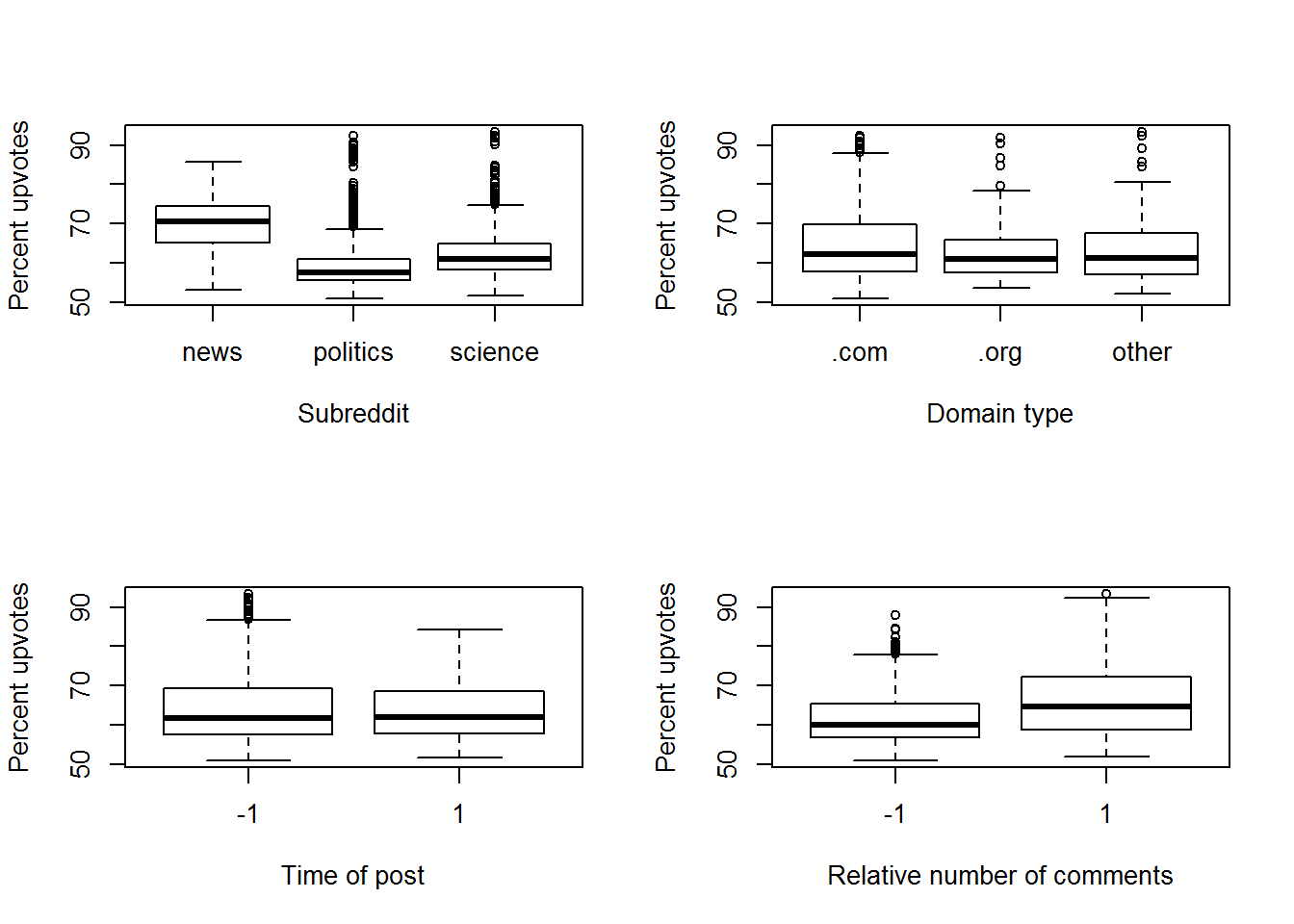Fig. 5 Box plots showing the main effects for the $$2^{2}*3^{2}$$ full factorial design.

However, upon plotting a histogram of the response variable, percent_upvotes, we can see that the distribution is strongly skewed to the right (Fig. 6). This makes sense in retrospect, since the raw data only includes “Top Posts” on Reddit, rather than all posts. Therefore, posts with a lower percent of upvotes were essentially culled from the dataset.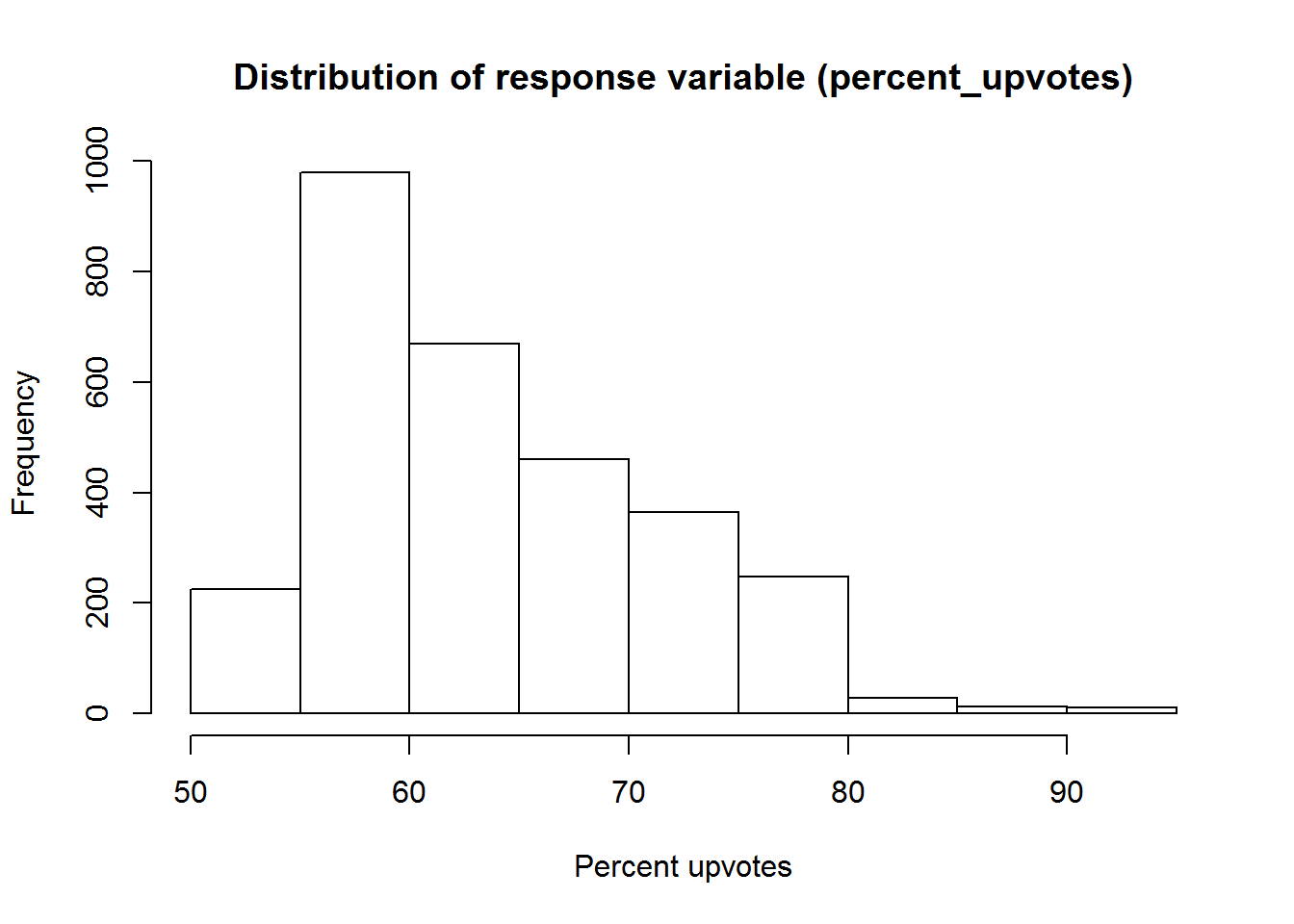Fig. 6 Histogram showing the strongly skewed distribution of the response variable.

### Testing

Upon performing ANOVA on a linear model explained by the main effects, it was found that none of the main effects were statistically significant. Part of the reason could be that the 3-level factors were not ordinal, and therefore upon decomposition into two 2-level factors, the middle factor convoluted any difference that might have existed in a $$2^{2}*3^{2}$$ design. Additionally, there were only 8 runs conducted in this $$2_{III}^{6-3}$$ fractional factorial design. Assuming that the effect sizes are weak, such a small sample is unlikely to produce significant results. Therefore, the fractional factorial design is probably not the best design for this type of dataset.

# perform ANOVA on the linear model looking only at main effects
MyAOV <- aov(lm(results$as.vector.response.percent_upvotes. ~ (results$SR1 + results$SR2 + results$DT1 + results$DT2 + results$time_code + results$comments_code))) summary(MyAOV) ## Df Sum Sq Mean Sq F value Pr(>F) ## results$SR1            1   6.20    6.20   0.217  0.722
## results$SR2 1 65.66 65.66 2.300 0.371 ## results$DT1            1  76.13   76.13   2.666  0.350
## results$DT2 1 138.72 138.72 4.859 0.271 ## results$time_code      1   5.18    5.18   0.181  0.744
## results$comments_code 1 30.06 30.06 1.053 0.492 ## Residuals 1 28.55 28.55 ### Contingencies to the experimental design It should be noted that Reddit vote tallying, and subsequently the list of top posts, is subject to a number of rules and algorithms that Reddit engineers keep secret to prevent people from gaming the voting system. Some of these rules have artificially deflated top-voted posts over the past 11 years. Recently, on Dec. 6, 2016, Reddit instituted a new voting system that no longer suppresses the top scores.2 The dataset used in this analysis applies to the voting system prior to this changeover. Because the Reddit tallying algorithms affect the level of exposure a post sees, these algorithms affect what shows up on the front page of Reddit. ### Summary of relevant theory Given that we performed a fractional factorial design to save the costs of running an experiment, we lost some information due to aliasing. Appendix X of Montgomery provides a list of alias relationships for $$2^{k-p}$$ fractional factorial designs.1 Specifically, for a $$2_{III}^{6-3}$$ design, we are given the following information about the defining relation and aliases: Design generators: $$D=AB$$, $$E=AC$$, $$F=BC$$ Defining relation: $$I=ABD=ACE=BCDE=BCF=ACDF=ABEF=DEF$$ Aliases: $$A=BD=CE=CDF=BEF$$ $$B=AD=CF=CDE=AEF$$ $$C=AE=BF=BDE=ADF$$ $$D=AB=EF=BCE=ACF$$ $$E=AC=DF=BCD=ABF$$ $$F=BC=DE=ACD=ABE$$ $$CD=BE=AF=ABC=ADE=BDF=CEF$$ The aliasing structure can also be viewed using the aliasprint function in FrF2. # show aliasing using aliasprint() function aliasprint(plan) ##$legend
##  A=SR1           B=SR2           C=DT1           D=DT2
##
## $main ##  A=BD=CE B=AD=CF C=AE=BF D=AB=EF E=AC=DF F=BC=DE ## ##$fi2
##  AF=BE=CD

The resolution is determined by the length of the shortest word in the defining relation, I. Here, the shortest words (ABD, ACE, BCF, and DEF) have three letters, hence Resolution III. By allowing more trial runs, we can increase the length of the defining relations and increase the resolution.

By examining the alias structure, we can see that all two-factor interactions are aliased with the main effects for this Resolution III design. Therefore, if we expect to have interactions between variables, it would be wise to perform more trial runs in the design (16 or 32) to achieve a higher resolution (IV or V, respectively).

## 5. Appendices

### Raw data

The datasets for all subreddits can be found at https://github.com/umbrae/reddit-top-2.5-million. The chosen datasets for this study were r/science, r/politics, and r/news, which are easily searchable from the URL and can be downloaded as .CSV files. In this report, the RCurl library was used to retrieve the raw .CSV files directly from their respective URLs.

### Complete and documented R code

# Project 3: Fractional Factorial Designs
# ISYE 6020
# Mike Deagen

# Objective: Explore polarization (in terms of % upvotes) for top posts in various subreddits: r/science, r/politics, and r/news

# include RCurl library to be able to retrieve .CSV from a URL
library(RCurl)
# FrF2 library must be installed and loaded
library(FrF2)
# dplyr library must be installed and loaded
library(dplyr)

# obtain raw data from online repository
science_data <- getURL('https://raw.githubusercontent.com/umbrae/reddit-top-2.5-million/master/data/science.csv', ssl.verifyhost=FALSE, ssl.verifypeer=FALSE)
politics_data <- getURL('https://raw.githubusercontent.com/umbrae/reddit-top-2.5-million/master/data/politics.csv', ssl.verifyhost=FALSE, ssl.verifypeer=FALSE)
news_data <- getURL('https://raw.githubusercontent.com/umbrae/reddit-top-2.5-million/master/data/news.csv', ssl.verifyhost=FALSE, ssl.verifypeer=FALSE)
# import raw data as data frames

# drop columns NOT of interest
DropColumns <- function(x){
x <- subset(x, select = -c(id, title, author, permalink, selftext, link_flair_text, over_18, thumbnail, edited, link_flair_css_class, author_flair_css_class, is_self, name, url, distinguished, subreddit_id))
return(x)
}
# apply the DropColumns function to each dataframe
science_df <- DropColumns(science_df)
politics_df <- DropColumns(politics_df)
news_df <- DropColumns(news_df)

# add column with subreddit name
science_df[,"subreddit_name"] <- c("science")
politics_df[,"subreddit_name"] <- c("politics")
news_df[,"subreddit_name"] <- c("news")

# print the header of each data frame

# join all datasets together
all_data <- rbind(science_df, politics_df, news_df)

# create column with NORMALIZED comments (as a percent of total votes)
all_data$percent_comments <- (100*all_data$num_comments/(all_data$ups + all_data$downs))

# convert domain column to CHAR type
all_data$domain <- as.character(all_data$domain)

# create column with DOMAIN TYPE (.org, .com, or other)
for(i in 1:nrow(all_data)) {

if (endsWith(all_data[i,'domain'],'.org')) {
all_data[i,'domain_type'] <- c('.org')
}
else if (endsWith(all_data[i,'domain'],'.com')) {
all_data[i,'domain_type'] <- c('.com')
}
else {
all_data[i,'domain_type'] <- c('other')
}
}

all_data$percent_upvotes <-(100*all_data$ups/(all_data$ups + all_data$downs))

# view structure, head, and tail of the dataset
str(all_data)
tail(all_data)

# define cutoff for comments HIGH/LOW
cutoff_comments <- median(all_data$percent_comments) # define cutoff time cutoff_time <- median(all_data$created_utc)

# determine EXPERIMENT CODE (0-35) based on factor levels
for(i in 1:nrow(all_data)) {
# initialize coded 0/1 for time and comments cutoffs
all_data[i,'time_code'] <- -1

# initialize exp_code as zero
all_data[i,'exp_code'] <- 0

# SORT BY SUBREDDIT NAME
# if subreddit_name is 'politics', add 12
if (all_data[i,'subreddit_name']=='politics') {
all_data[i,'exp_code'] <- all_data[i,'exp_code'] + 12
}
# if subreddit_name is 'politics', add 24
else if (all_data[i,'subreddit_name']=='news') {
all_data[i,'exp_code'] <- all_data[i,'exp_code'] + 24
}

# SORT BY DOMAIN TYPE
# if domain_type is '.com', add 4
if (all_data[i,'domain_type']=='.com') {
all_data[i,'exp_code'] <- all_data[i,'exp_code'] + 4
}
# if domain_type is 'other', add 8
else if (all_data[i,'domain_type']=='other') {
all_data[i,'exp_code'] <- all_data[i,'exp_code'] + 8
}

# SORT BY TIME CUTOFF (and code -1/1 based on designation)
# if created_utc is GREATER THAN cuttoff_time, add 2
if (all_data[i,'created_utc'] > cutoff_time) {
all_data[i,'exp_code'] <- all_data[i,'exp_code'] + 2
all_data[i,'time_code'] <- 1
}

# SORT BY COMMENTS CUTOFF (and code -1/1 based on designation)
all_data[i,'exp_code'] <- all_data[i,'exp_code'] + 1
}
}

# check spread of exp codes
hist(all_data$exp_code, breaks = 36, xlab="Experiment Code (0-35)", main = "Confirmation that all factor-level combinations exist") # determine the least-occurring exp_code(s), which will limit the number of possible replicates exp_table <- table(all_data$exp_code)
as.numeric(names(exp_table[exp_table == min(exp_table)]))

# determine the maximum number of replicates, which is the number of occurrences of the least-occurring codes
min(exp_table)

# set appropriate columns as factors
all_data$subreddit_name <- as.factor(all_data$subreddit_name)
all_data$domain_type <- as.factor(all_data$domain_type)
all_data$time_code <- as.factor(all_data$time_code)
all_data$comments_code <- as.factor(all_data$comments_code)

# examine the structure of the data frame
str(all_data)

# set RNG seed (for reproducible results)
set.seed(1)

# create the sample
my_sample <- all_data %>% group_by(exp_code) %>% sample_n(size = min(exp_table))

tail(my_sample)

# create a new dataset for breaking down 3-level factors into two 2-level factors each (and remove old exp_code column)
all_data2 <- subset(all_data, select = -c(exp_code))

# toggle variables that will determing splitting of middle of 3-level into (-1,1) or (1,-1)
SR_toggle <- TRUE
DT_toggle <- TRUE

for(i in 1:nrow(all_data2)) {

if (all_data2[i,'domain_type']=='.org') {
all_data2[i,'DT1'] <- -1
all_data2[i,'DT2'] <- -1
}
else if (all_data[i,'domain_type']=='.com') {
if (DT_toggle) {
all_data2[i,'DT1'] <- 1
all_data2[i,'DT2'] <- -1
}
else {
all_data2[i,'DT1'] <- -1
all_data2[i,'DT2'] <- 1
}
DT_toggle <- !DT_toggle
}
else {
all_data2[i,'DT1'] <- 1
all_data2[i,'DT2'] <- 1
}

if (all_data2[i,'subreddit_name']=='news') {
all_data2[i,'SR1'] <- -1
all_data2[i,'SR2'] <- -1
}
else if (all_data[i,'subreddit_name']=='politics') {
if (SR_toggle) {
all_data2[i,'SR1'] <- 1
all_data2[i,'SR2'] <- -1
}
else {
all_data2[i,'SR1'] <- -1
all_data2[i,'SR2'] <- 1
}
SR_toggle <- !SR_toggle
}
else {
all_data2[i,'SR1'] <- 1
all_data2[i,'SR2'] <- 1
}
}

# The 2^6 design now has 64 possible experiment codes
# Apply similar sorting structure as above, this time using DT1 and DT2 in place of domain_type and SR1 and SR2 in place of subreddit_name
# determine EXPERIMENT CODE (0-63) based on factor levels
for(i in 1:nrow(all_data2)) {
# initialize exp_code as zero
all_data2[i,'exp_code'] <- 0

# SORT BY SR1
# if SR1 is +1, add 32
if (all_data2[i,'SR1']=='1') {
all_data2[i,'exp_code'] <- all_data2[i,'exp_code'] + 32
}

# SORT BY SR2
# if SR2 is +1, add 16
if (all_data2[i,'SR2']=='1') {
all_data2[i,'exp_code'] <- all_data2[i,'exp_code'] + 16
}

# SORT BY DT1
# if DT1 is +1, add 8
if (all_data2[i,'DT1']=='1') {
all_data2[i,'exp_code'] <- all_data2[i,'exp_code'] + 8
}

# SORT BY DT2
# if DT2 is +1, add 4
if (all_data2[i,'DT2']=='1') {
all_data2[i,'exp_code'] <- all_data2[i,'exp_code'] + 4
}

# SORT BY TIME CUTOFF (coded -1/1 based on designation)
# if time_code is +1, add 2
if (all_data2[i,'time_code']=='1') {
all_data2[i,'exp_code'] <- all_data2[i,'exp_code'] + 2
}

# SORT BY COMMENTS CUTOFF (coded -1/1 based on designation)
all_data2[i,'exp_code'] <- all_data2[i,'exp_code'] + 1
}
}

# check spread of exp codes for all_data2
hist(all_data2$exp_code, breaks = 64, xlab="Experiment Code (0-63)", main = "Confirmation that all factor-level combinations exist") # determine the least-occurring exp_code(s), which will limit the number of possible replicates exp_table2 <- table(all_data2$exp_code)
as.numeric(names(exp_table2[exp_table2 == min(exp_table2)]))

# determine the maximum number of replicates, which is the number of occurrences of the least-occurring codes
min(exp_table2)

# create the sample
my_sample2 <- all_data2 %>% group_by(exp_code) %>% sample_n(size = min(exp_table2))

# set appropriate columns as factors, and return subreddit_name and domain_type to characters
all_data2$SR1 <- as.factor(all_data2$SR1)
all_data2$SR2 <- as.factor(all_data2$SR2)
all_data2$DT1 <- as.factor(all_data2$DT1)
all_data2$DT2 <- as.factor(all_data2$DT2)
all_data2$time_code <- as.factor(all_data2$time_code)
all_data2$comments_code <- as.factor(all_data2$comments_code)
all_data2$subreddit_name <- as.character(all_data2$subreddit_name)
all_data2$domain_type <- as.character(all_data2$domain_type)

tail(my_sample2)

# create fractional factorial design using FrF2 package
plan <- FrF2(8, 6, factor.names = c("SR1", "SR2", "DT1", "DT2", "time_code", "comments_code"))
mydesign <- as.data.frame(plan)

# create a new row in mydesign with the experiment code corresponding to the factor level sorting algorithm used earlier
for(i in 1:nrow(mydesign)) {
# initialize exp_code as zero
mydesign[i,'exp_code'] <- 0

# SORT BY SR1
# if SR1 is +1, add 32
if (mydesign[i,'SR1']=='1') {
mydesign[i,'exp_code'] <- mydesign[i,'exp_code'] + 32
}

# SORT BY SR2
# if SR2 is +1, add 16
if (mydesign[i,'SR2']=='1') {
mydesign[i,'exp_code'] <- mydesign[i,'exp_code'] + 16
}

# SORT BY DT1
# if DT1 is +1, add 8
if (mydesign[i,'DT1']=='1') {
mydesign[i,'exp_code'] <- mydesign[i,'exp_code'] + 8
}

# SORT BY DT2
# if DT2 is +1, add 4
if (mydesign[i,'DT2']=='1') {
mydesign[i,'exp_code'] <- mydesign[i,'exp_code'] + 4
}

# SORT BY TIME CUTOFF (coded -1/1 based on designation)
# if time_code is +1, add 2
if (mydesign[i,'time_code']=='1') {
mydesign[i,'exp_code'] <- mydesign[i,'exp_code'] + 2
}

# SORT BY COMMENTS CUTOFF (coded -1/1 based on designation)
eligibledata <- all_data2[is.element(all_data2$exp_code, mydesign$exp_code),]
hist(eligibledata$exp_code, breaks = 64, xlab="Experiment Code (0-63)", main = "Eligible data for sampling") # create a sample of the eligible data fractionaldata <- eligibledata %>% group_by(exp_code) %>% sample_n(size = 1) # show the values of the response variable (percent_upvotes) for each corresponding exp_code response <- fractionaldata[,c("exp_code", "percent_upvotes")] # re-arrange rows of the response variables so that the exp_code matches up with the mydesign dataframe response <- response[match(as.vector(mydesign$exp_code), response$exp_code),] # add column with the response variable to the FrF2 design results <- add.response(plan, as.vector(response$percent_upvotes))
MyAOV <- aov(lm(results$as.vector.response.percent_upvotes. ~ (results$SR1 + results$SR2 + results$DT1 + results$DT2 + results$time_code + results$comments_code))) summary(MyAOV) # show aliasing using aliasprint() function aliasprint(plan) #EXPLORATORY DATA ANALYSIS # boxplots of response variables for full dataset par(mfrow=c(2,2)) boxplot(percent_upvotes~subreddit_name, all_data, xlab="Subreddit", ylab = "Percent upvotes") boxplot(percent_upvotes~domain_type, all_data, xlab="Domain type", ylab = "Percent upvotes") boxplot(percent_upvotes~time_code, all_data, xlab="Time of post", ylab = "Percent upvotes") boxplot(percent_upvotes~comments_code, all_data, xlab="Relative number of comments", ylab = "Percent upvotes") # generate histogram of the response variable, percent_upvotes hist(all_data$percent_upvotes, main="Distribution of response variable (percent_upvotes)", xlab="Percent upvotes")